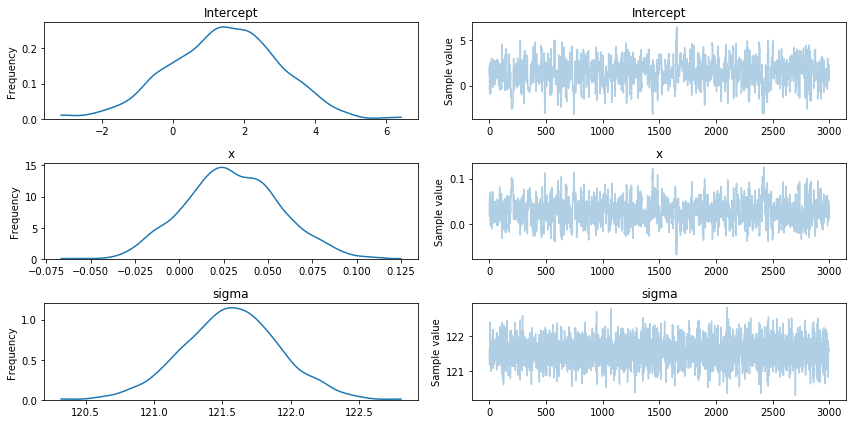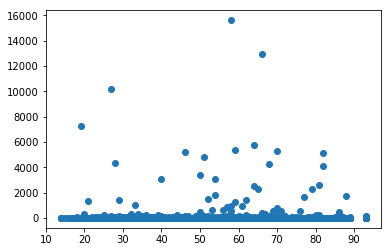### Bayesian Regression with PYMC3 in PythonOkay, so normally I do frequentist statistics. Most of my training has been in that realm. However, I do recognize that bayesian is really the way to go. The idea of updating a prior is really appealing to me. The only problem that I have ever had with it, is that I really haven’t had a good way to do bayesian statistics until I got into doing most of my work in python. There is a really cool library called pymc3.

So I want to go over how to do a linear regression within a bayesian framework using pymc3. Making inferences based off of what we learned from the data. And other non-sense related to probablistic programming.

So here’s what we are going to cover:

• The basic math of baesian inference
• Setting up a linear regression within a bayesian framework
• Writing the code in python
• spitting out answers

## Obligatory “What is Bayes Rule? And Why do We Care?” Section

Okay, so Baye’s rule is basically a way of encoding our prior beliefs about how the world works onto the world that we observe. That’s probably a terrible definition, but it is the one rattling around in my brain that doesn’t involve any math. Essentially, we get to combine what we already know with evidence out in the world to tell us about the state of the world.

Here’s an example. Let’s say that there is this rare disease out there, that randomly gets contracted by 1 in 10,000 people. In other words, you have a 0.01% chance of getting this disease. Not too shaby. And lucky enough there is an inexpensive test that correctly identifies people with this disease 99% of the time, and if you don’t have the disease it also correctly says that you do not have the disease 99% of the time. You take the test and it comes back positive. How worried should you be?

Well, let’s think about this logically for a moment. We know that only 1 in 10,000 people get this disease. So let’s say there are 10,000 people. 9,999 of them do not have the disease but 1% of these people will get a positive result. So about 101 people get a positive result, even though only 1 of them actually has the disease. That means that even with a positive result, you only have 1 in 101 chance of actually having the disease (or roughly a 1% chance).

Baye’s rule is the formal mathematical description of this idea. And here it is in its full glory:$p(X|Y)=\dfrac{p(Y|X)p(X)}{p(Y)}$

It looks so simple. And in fact, it is simple. The formula only requires knowledge of a few probability distributions. But in practice the denominator on the right hand side usually means that we are going to be computing a lot of really computationally heavy integrals. For this reason, bayesian statistics was abandoned for many years. In some sense it flows more naturally out of probability theory. If we only had things that were good at computing lots and lots of numbers to make this type of problem tractable. Enter modern computers.

Computers are really fast at making computations. In fact, my crappy old laptop that I am writing this post on, can do some decent bayesian statistics, like the bayesian regression that we are about to do.

So let’s break down this formula. P(Y|X): We typically think of this as our likelihood function of our data Y given parameters X. P(X) is our prior beliefs about parameter X. Finally, P(Y) is the probability of our data. This is typically computed using some crazy calculus on things without closed form solutions. Which is the whole “boo!” moment that we will use pymc3 to solve.

## Okay enough theory show me some code!

No code, not yet! Here’s what we need to know to do Bayesian Regression. Normally, we think of a regression like this:$Y=Xb+e$

And e is the error that is normally distributed. If you stop and think about it, this doesn’t make a lot of sense. You have a random variable that you are treating as if it is composed of a deterministic thing, and a random thing and smashing them together, so that Y looks random, but really is mostly not random.

And then if e doesn’t follow all of your rules, it completely invalidates your results. Check out these posts for examples of how having an e that isn’t normally distributed can ruin your day in a time series setting. Wouldn’t it be nice if we could just assume that Y is indeed a random variable 100% and not bother with this decomposition stuff. Well, if you do you will firmly live in bayesian land.

So we assume that:$Y~N(Xb,\sigma^{2})$

With priors:$b~N(\mu_b,\sigma_{b}^{2}$$\sigma^{2}~HalfCauchy(\beta)$

And so if we have data for X and Y we have everything that we need to do a bayesian linear regression. This will allow you to get full posterior distributions for your parameters in b. I think that’s pretty cool, because not only have you eliminated a lot of your problems by assuming that Y is a fully random variable, you can say how confident you are that a coefficient is positive. So let’s grab some data and start coding.

## Finally, I get to see some code!

Believe it or not the information that I provided in the last section is everything that we need to code a linear regression in pymc3. We just need to grab some data to make it work.

So the dataset that we are going to use is the American Housing Survey: Housing Affordability Data System dataset from 2013. Go ahead and download this data, and then unzip it and save it on your computer. We’ll be looking at this dataset for the remainder of this blog post.

What we’re interested in is how housing burden changes with age. AGE1 contains the age of the head of household. And BURDEN is a variable that tells us how big are housing expenses relative to income. For simplicity, we’ll just focus on these two variables. What we want to know is, does the burden of housing get easier as we age? In particular we want to know whether the slope coefficient is negative, and since we’re in a bayesian framework, what is the probability that it is negative?

So let’s start off with some prerequisites, we’ll import the libraries that we need and the data. We’ll also do some minor data cleaning because the documentation says that missing values are coded with a negative number, we’re going to drop them.

import pandas as pd
import pymc3
import matplotlib.pyplot as plt

df=pd.read_csv('/home/ryan/Documents/thads2013n.txt',sep=',')
df=df[df['BURDEN']>0]
df=df[df['AGE1']>0]


Alright so that was pretty simple. Now let’s build the model that we discussed above. It isn’t much to look at, so let’s do a scatter plot and see what the data looks like.

plt.scatter(df['AGE1'],df['BURDEN'])
plt.show()


And here is the result:It looks like the data has some ouliers where the housing burden is astronomically high, easily over 10 times income.

For now, we won’t worry too much about that. Here is the code to build and run our model:

with pm.Model() as model:
# Define priors
sigma = pm.HalfCauchy('sigma', beta=10, testval=1.)
intercept = pm.Normal('Intercept', 0, sd=20)
x_coeff = pm.Normal('x', 0, sd=20)

# Define likelihood
likelihood = pm.Normal('y', mu=intercept + x_coeff * df['AGE1'],
sd=sigma, observed=df['BURDEN'])

# Inference!
trace = pm.sample(3000)
pm.traceplot(trace)
plt.show()


It looks exactly like our model above, except that we have an extra beta for the intercept that is normally distributed as well. The last line is what actually runs the model for us. Now that our model is trained we can go on to doing some inferential work. Go ahead and do something else while this is running. On an older laptop, like mine, this can take a fair amount of time. Typically, you will want to do these calculations in the cloud on a GPU, but we’ll just let it run. It took 47 minutes to run on my laptop, did I mention that it is ancient. After it finishes running you should see something like this:What the trace variable contains is the posterior distribution for our parameter values. You can see that we have our slope and intercept posterior distribution plus the standard deviation for the regression.

But like I was wondering at the beginning, does housing burden decrease with age? My thought was that perhaps it did. As people get more established, their housing costs would go down relative to income. This would amount to a negative slope coefficient on the age variable. From the figure above, I can see that the posterior distribution for the slope parameter, and it isn’t looking so great for my hypothesis that it should be negative, it looks like it peaks around 0.025. In fact, if I run the following code, I can find out what the exact probability that the slope coefficient is negative.

print(np.mean([1 if obj<0 else 0 for obj in trace['x']]))


When I ran it, I got about a 13.8% probability that the coefficient is negative. I don't think that is good enough evidence for me. Alas, it looks like I was wrong. Your run should produce a similar result, but because of the simulation for the integral results may vary (but not by much).

And there it is, bayesian linear regression in pymc3. It wasn't so bad. In fact, pymc3 made it downright easy. That's why python is so great for data analysis. Let me know what you think about bayesian regression in the comments below!

As always, here is the full code for everything that we did:

import pandas as pd
import pymc3 as pm
import matplotlib.pyplot as plt
import numpy as np

df=pd.read_csv('/home/ryan/Documents/thads2013n.txt',sep=',')
df=df[df['BURDEN']>0]
df=df[df['AGE1']>0]

plt.scatter(df['AGE1'],df['BURDEN'])
plt.show()

with pm.Model() as model:
# Define priors
sigma = pm.HalfCauchy('sigma', beta=10, testval=1.)
intercept = pm.Normal('Intercept', 0, sd=20)
x_coeff = pm.Normal('x', 0, sd=20)

# Define likelihood
likelihood = pm.Normal('y', mu=intercept + x_coeff * df['AGE1'],
sd=sigma, observed=df['BURDEN'])

# Inference!
trace = pm.sample(3000)
pm.traceplot(trace)
plt.show()
print(np.mean([1 if obj<0 else 0 for obj in trace['x']]))